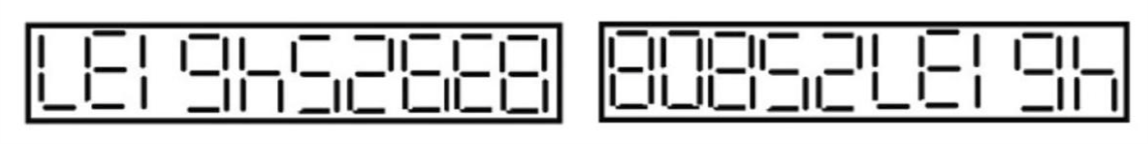# Sunday Times Teaser 2925 – “The Eternal Triangle” — Go Figure!

### by Stephen Hogg

#### Published October 14 2018 (link)From my desk I saw the above when Leigh and Bob put 8392546137 and 4613725808 into two of the identical calculators used by my class. Everyone was told to press “clear”, then enter their unique three-figure pupil number (between 100 and 999) and divide this by the total number of display segments seen in it. If the result was a whole number they were to repeat the process with that result and its segment count (and so on until a decimal fraction part arose). Leigh, Bob and Geb each made four “divisions” this way. Using their three initial values as the sides of a triangle (Bob’s shortest, Geb’s longest), I told them to find its area.

What was Leigh’s number?

From → Uncategorized